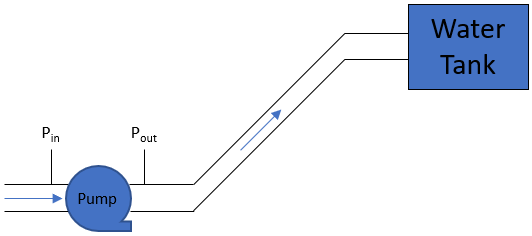## Pressurized Pump Power

In the below figure, a water pump with 90% efficiency is pushing fluid upward at a 5 cubic meters per second flow rate. If the measured inlet and outlet pressures are measured at 500 kPa and 150 kPa respectively, what is the pump’s minimum required power? Assume the specific weight of water is 9.8 kN/m^3.Hint
The pump power equation:
$$\dot{W}=\frac{Q\gamma h}{\eta_t}$$$where $$Q$$ is the volumetric flow, $$h$$ is the fluid head needed to be lifted, $$\eta_t$$ is the total efficiency ( $$\eta_{pump} \times \eta_{motor}$$ ), $$\dot W$$ is the power, and $$\gamma$$ is the specific weight of the fluid. Hint 2 How pressure changes with elevation in a fluid can be expressed as: $$\Delta P=\gamma \times \Delta h$$$
where $$\Delta P$$ is the change is pressure, $$\Delta h$$ is the change in height, and $$\gamma$$ is the fluid’s specific weight ( $$density \times gravity$$ ).
The pump power equation:
$$\dot{W}=\frac{Q\gamma h}{\eta_t}$$$where $$Q$$ is the volumetric flow, $$h$$ is the fluid head needed to be lifted, $$\eta_t$$ is the total efficiency ( $$\eta_{pump} \times \eta_{motor}$$ ), $$\dot W$$ is the power, and $$\gamma$$ is the specific weight of the fluid. Remember, how pressure changes with elevation in a fluid can be expressed as: $$\Delta P=\gamma \times \Delta h$$$
where $$\Delta P$$ is the change is pressure, $$\Delta h$$ is the change in height, and $$\gamma$$ is the fluid’s specific weight ( $$density \times gravity$$ ). Solving for fluid head:
$$\Delta h=\frac{\Delta P}{\gamma}=\frac{500kPa-150kPa}{9.8kN/m^3}=\frac{350,000N/m^2}{9,800N/m^3}=35.7\:m$$$Therefore, $$\dot{W}=\frac{(5m^3)(9.8kN)(35.7m)}{(sec)(m^3)(0.9)}=\frac{1,749.3kN\cdot m}{0.9(sec)}=1,944\:kW$$$
1,944 kW